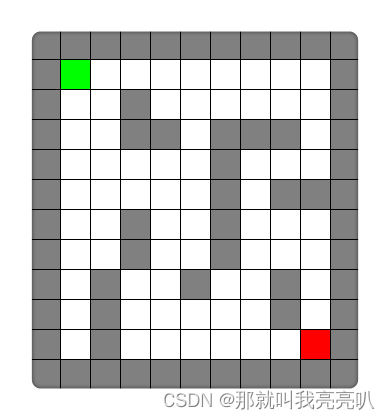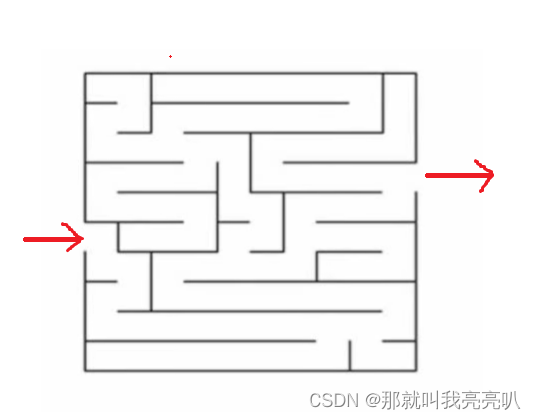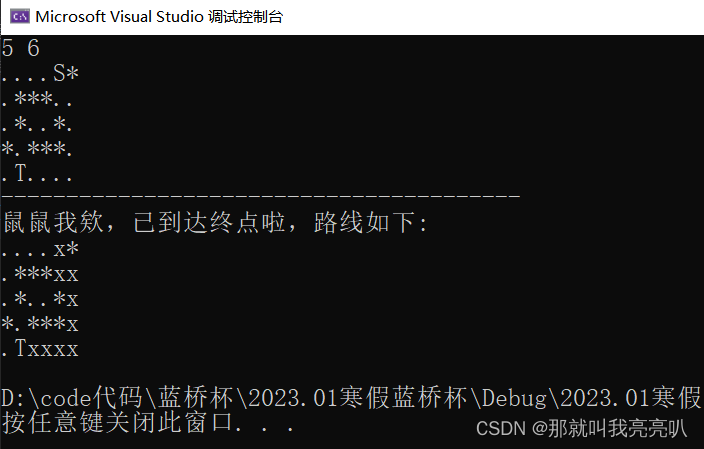# 【C++算法】dfs深度优先搜索(上) ——【全面深度剖析+经典例题展示】

💃🏼 本人简介：男
👶🏼 年龄：18
📕 ps:七八天没更新了欸，这几天刚搞完元宇宙，上午一直练🚗，下午背四级单词和刷题来着，还在忙一些学弟学妹录制视频和准备开学一些事，一直没空出时间来，等 20号练完车，也马上开学了QAQ。不过今天倒是空出来一些时间，恰好这几天学到了dfs，原理和例题都很棒，谨以此篇作为学后的回顾总结！

TabLayout

yum

# 1. dfs算法原理

## 1.1 dfs思想

• 深度优先搜索，简称dfs，简单讲就是一个搜索算法。
• 深搜是按照`深度优先`的方式进行搜索，通俗来讲就是`一条路走到黑``不撞南墙不回头`
• 注意：这里的`搜索`并不是我们平时在文件上或网络上查找信息，而是通过一种`穷举`的方式，把所有可行的方案都列举出来，不断去尝试，直到找到问题的解。
• 具体来讲，dfs可以将“问题状态空间”看做一棵搜索树，深度优先就是从`树根一直往下搜，遇到不可解就回溯，往其它方向继续向下扩展`，像子集和和全排列问题，还有N皇后问题都可以深度优先搜索算法解决，它是一种暴力解决NP问题的非常直观的方法。
• 总的来说：DFS 用于找所有解的问题，它的空间效率高，而且找到的不一定是最优解，必须记录并完成整个搜索，故一般情况下，深搜需要非常高效的剪枝（剪枝的概念请百度）。

## 1.2 与递归区别

• 深搜是一种算法，注重的是思想；而递归是一种基于编程语言的实现方式。
• 深搜可以用递归实现，也就是说递归是我们用计算机编程语言实现深搜算法的手段。

## 1.3 举例说明

j2eeKruskal

# 2. 经典例题——迷宫游戏web3

logging

## 2.1 题干信息

• 我们用一个二维字符数组来表示图画的迷宫。
``````S**.
....
***T
``````
• 其中`S`表示起点，`T`表示终点，`*`表示墙壁，`.`表示平地。你需要从`S`出发走到`T`，每次只能向上下左右相邻的位置移动一位，不能走出地图，也不能穿过墙壁，每个点只能通过一次。用`x`表示你所要走的路线。

## 2.2 整体思路

• 我们从起点S开始，每走一步需要对上下左右一个方向一个方向地尝试，如果沿着某个方向不能走到终点，我们就要原路返回，继续尝试其他方向，直到走出迷宫。这是一种最朴素的走迷宫方式，虽然效率也许比较低，但如果迷宫有解，就一定能走出终点。
• 上面说的这种走法，就对应着今天学习的dfs算法。首先找到起点s,走到每个点时，按照左、下、右、上的顺序尝试。每走到下一个点以后，我们把这个点当做起点S，继续按顺序尝试。如果某个点上下左右四个方向都尝试过，便回到走到这个点之前的点，这一步我们称之为回溯。继续尝试其他方向。直到所有点都尝试过上下左右四个方向。
• 这就好比你自己去走这个迷宫，你也要一个方向一个方向的尝试着走，如果这条路不行就回头，尝试下一条路，dfs 的思想和我们直观的想法很类似。只不过，接下来我们需要用程序来完成这个过程。

## 2.3 细分拆解

``````#include<iostream>
#include<stdio.h>
using namespace std;
int n, m;
char pos;	   //判断走没走过
bool trace;  //显示路径

int main() {
//输入地图
cin >> n >> m;
for (int i = 1; i <= n; i++) {
for (int j = 1; j <= m; j++) {
cin >> pos[i][j];
}
}
return 0;
}

``````

### ①判断迷宫终点，记录所走路径

• 首先确定边界条件，当走到字符`T`时，我们找到了终点，从而结束搜索。所以边界条件判断为`pos[x][y] == 'T'`
• 其次，为了防止走回头路，我们需要标记当前这个路径已走过，即当前这个点已走过，所以我们需要用`trace[x][y]`数组来做标记，为了显示出路径，走过的点我们用字符`x`表示。
``````bool dfs(int x, int y) {
if (pos[x][y] == 'T') { //找到终点，返回true
return true;
}
trace[x][y] = 1;		//若找不到，则trace数组标记为1表示已走过
pos[x][y] = 'x';		//用pos显示最后的路径
}
``````

### ②完善搜索与回溯，处理数组边界

• 结束操作处理好后，就要开始真正的搜索了。假设现在我们坐标为`(x, y)`,分别遍历该坐标的上下左右位置，选择好依次进行方向的顺序后，一个方向一个方向进行访问，如果某一方向能走到终点，则返回`true`
• 在上下左右遍历时，我们要考虑数组元素是否越界，此时我们就需要一个`bool`类型的`check_in()`函数进行判断。
• 注意：判断移动后位置能走的3个条件【缺一不可】
• ①没越界，在地图内；
• ②这个位置不是障碍物*，可以走到；
• ③该位置之前没走过
``````bool check_in(int x, int y) {	//	判断数组是否越界
return (x > 0 && x <= n && y > 0 && y <= m); //这里表示的是如果()里为真，则返回true，否则返回false
}

bool dfs(int x, int y) {
if (pos[x][y] == 'T') { //找到终点，返回true
return true;
}
trace[x][y] = 1;		//若上下左右都找不到，则trace数组标记为1表示已走过
pos[x][y] = 'x';		//用pos显示最后的路径

int tx = x - 1, ty = y; //假设先往上走
if (check_in(tx, ty) && pos[tx][ty] != '*' && !trace[tx][ty]) { //能移动到该位置的条件有三个：①没越界，在地图内； ②这个位置不是障碍物*，可以走到； ③该位置之前没走过
if (dfs(tx, ty)) {	//对移动后位置进行判断是不是终点，如果是，返回true，如果不是，在对其上下左右判断。
return true;
}
}

tx = x, ty = y - 1; //如果往上走行不通，则选择向左走
if (check_in(tx, ty) && pos[tx][ty] != '*' && !trace[tx][ty]) {
if (dfs(tx, ty)) {
return true;
}
}

tx = x + 1, ty = y; //如果往左走行不通，则选择向下走
if (check_in(tx, ty) && pos[tx][ty] != '*' && !trace[tx][ty]) {
if (dfs(tx, ty)) {
return true;
}
}

tx = x , ty = y + 1; //如果往下走行不通，则选择向右走
if (check_in(tx, ty) && pos[tx][ty] != '*' && !trace[tx][ty]) {
if (dfs(tx, ty)) {
return true;
}
}

pos[x][y] = '.';	//	如果一个位置的上下左右都走不了，则取消该位置的路径进行回溯，回溯过程把之前已标记的'x'改回'.'
trace[x][y] = 0;//只找可行路线的话，trace可改可不改。但如果找全部解，则需要恢复
return false;
}
``````

### ③找寻迷宫起点，打印结束路径

• 我们费心费力地把迷宫主体的dfs的函数写完了，但我们该从哪开始呢？结束条件如何设置呢？
``````int main() {
//输入地图
cin >> n >> m;
for (int i = 1; i <= n; i++) {
for (int j = 1; j <= m; j++) {
cin >> pos[i][j];
}
}
cout << "----------------------------------------" << endl;

//找寻起点
int x , y ; //定义x，y用于保存迷宫起点时的位置
for (int i = 1; i <= n; i++) {
for (int j = 1; j <= m; j++) {
if (pos[i][j] == 'S') {
x = i, y = j;
}
}
}
if (dfs(x, y)) { //如果能找到终点，则打印迷宫显示其路径
cout << "鼠鼠我欸，已到达终点啦，路线如下: " << endl;
for (int i = 1; i <= n; i++) {
for (int j = 1; j <= m; j++) {
cout << pos[i][j];
} cout << endl;
}
}
else {
cout << "鼠鼠我欸，走不出去啦awa" << endl;
}
return 0;
}
``````

## 2.4 总体代码展示

``````#include<iostream>
#include<stdio.h>
using namespace std;

int n, m;
char pos;	   //判断走没走过
bool trace;  //显示路径

bool check_in(int x, int y) {	//	判断数组是否越界
return (x > 0 && x <= n && y > 0 && y <= m); //这里表示的是如果()里为真，则返回true，否则返回false
}

bool dfs(int x, int y) {
if (pos[x][y] == 'T') { //找到终点，返回true
return true;
}
trace[x][y] = 1;		//若上下左右都找不到，则trace数组标记为1表示已走过
pos[x][y] = 'x';		//用pos显示最后的路径

int tx = x - 1, ty = y; //假设先往上走
if (check_in(tx, ty) && pos[tx][ty] != '*' && !trace[tx][ty]) { //能移动到该位置的条件有三个：①没越界，在地图内； ②这个位置不是障碍物*，可以走到； ③该位置之前没走过
if (dfs(tx, ty)) {	//对移动后位置进行判断是不是终点，如果是，返回true，如果不是，在对其上下左右判断。
return true;
}
}

tx = x, ty = y - 1; //如果往上走行不通，则选择向左走
if (check_in(tx, ty) && pos[tx][ty] != '*' && !trace[tx][ty]) {
if (dfs(tx, ty)) {
return true;
}
}

tx = x + 1, ty = y; //如果往左走行不通，则选择向下走
if (check_in(tx, ty) && pos[tx][ty] != '*' && !trace[tx][ty]) {
if (dfs(tx, ty)) {
return true;
}
}

tx = x , ty = y + 1; //如果往下走行不通，则选择向右走
if (check_in(tx, ty) && pos[tx][ty] != '*' && !trace[tx][ty]) {
if (dfs(tx, ty)) {
return true;
}
}

pos[x][y] = '.';	//	如果一个位置的上下左右都走不了，则取消该位置的路径进行回溯，回溯过程把之前已标记的'x'改回'.'
trace[x][y] = 0;//只找可行路线的话，trace可改可不改。但如果找全部解，则需要恢复
return false;
}
int main() {
//输入地图
cin >> n >> m;
for (int i = 1; i <= n; i++) {
for (int j = 1; j <= m; j++) {
cin >> pos[i][j];
}
}
cout << "----------------------------------------" << endl;
//找寻起点
int x , y ; //定义x，y用于保存迷宫起点时的位置
for (int i = 1; i <= n; i++) {
for (int j = 1; j <= m; j++) {
if (pos[i][j] == 'S') {
x = i, y = j;
}
}
}
if (dfs(x, y)) { //如果能找到终点，则打印迷宫显示其路径
cout << "鼠鼠我欸，已到达终点啦，路线如下: " << endl;
for (int i = 1; i <= n; i++) {
for (int j = 1; j <= m; j++) {
cout << pos[i][j];
} cout << endl;
}
}
else {
cout << "鼠鼠我欸，走不出去啦awa" << endl;
}
return 0;
}
``````

## 2.5 测试样例## 2.6 代码优化

• 前面提到，我们在对下一位置进行上下左右判断时，需要写四个主体相同的代码。为了减少代码量，我们不妨写一个循环，用一个二维数组依次表示四个方向，进而进行判断。
``````int dir = { { -1 , 0 } , { 0 , -1 } , { 1 ,  0 } , { 0 , 1 } };
//	按逆时针依次表示     向上	      向左		 向下	         向右
//第一个数表示x(行)变化，第二个表示y(列)变化
bool dfs(int x, int y) {
if (dfs(x, y) == 'T') {
return true;
}
trace[x][y] = 1;
pos[x][y] = 'x';
for (int i = 1; i <= 4; i++) {  //1、2、3、4依次表示上、左、下、右
int tx = x + dir[i]; //表示x加上第几个方向的第1个数，即行变化，
int ty = y + dir[i];  //表示x加上第几个方向的第2个数，即列变化，
if (check_in(tx, ty) && pos[tx][ty] != '*' && !trace[tx][ty]) {
if (dfs(tx, ty)) {
return true;
}
}
}
pos[x][y] = '.';	//	如果一个位置的上下左右都走不了，则取消该位置的路径进行回溯，回溯过程把之前已标记的'x'改回'.'
trace[x][y] = 0;//只找可行路线的话，trace可改可不改。但如果找全部解，则需要恢复
}
``````

sqlite

``````#include<iostream>
#include<stdio.h>
using namespace std;

int n, m;
char pos;	   //判断走没走过
bool trace;  //显示路径
int dir = { { -1 , 0 } , { 0 , -1 } , { 1 ,  0 } , { 0 , 1 } };
//	按逆时针依次表示     向上	      向左		 向下	         向右
//第一个数表示x(行)变化，第二个表示y(列)变化

bool check_in(int x, int y) {	//	判断数组是否越界
return x > 0 && x <= n && y > 0 && y <= m; //这里表示的是如果()里为真，则返回true，否则返回false
}

bool dfs(int x, int y) {
if (dfs(x, y) == 'T') {
return true;
}
trace[x][y] = 1;
pos[x][y] = 'x';
for (int i = 1; i <= 4; i++) {  //1、2、3、4依次表示上、左、下、右
int tx = x + dir[i]; //表示x加上第几个方向的第1个数，即行变化，
int ty = y + dir[i];  //表示x加上第几个方向的第2个数，即列变化，
if (check_in(tx, ty) && pos[tx][ty] != '*' && !trace[tx][ty]) {
if (dfs(tx, ty)) {
return true;
}
}
}
pos[x][y] = '.';	//	如果一个位置的上下左右都走不了，则取消该位置的路径进行回溯，回溯过程把之前已标记的'x'改回'.'
trace[x][y] = 0;//只找可行路线的话，trace可改可不改。但如果找全部解，则需要恢复

}

int main() {
//输入地图
cin >> n >> m;
for (int i = 1; i <= n; i++) {
for (int j = 1; j <= m; j++) {
cin >> pos[i][j];
}
}
cout << "----------------------------------------" << endl;
//找寻起点
int x , y ; //定义x，y用于保存迷宫起点时的位置
for (int i = 1; i <= n; i++) {
for (int j = 1; j <= m; j++) {
if (pos[i][j] == 'S') {
x = i, y = j;
}
}
}
if (dfs(x, y)) { //如果能找到终点，则打印迷宫显示其路径
cout << "鼠鼠我欸，已到达终点啦，路线如下: " << endl;
for (int i = 1; i <= n; i++) {
for (int j = 1; j <= m; j++) {
cout << pos[i][j];
} cout << endl;
}
}
else {
cout << "鼠鼠我欸，走不出去啦awa" << endl;
}
return 0;
}

``````

# 最后，感谢大家支持u （^ _ ^）

``````printf("点个赞吧*^*");
``````
``````cout << "收藏一下叭o_o";
``````
``````System.out.println("评论一下吧^_^");
``````
``````print("关注一下叭0-0")
``````